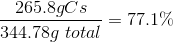# AP Chemistry : Mass

## Example Questions

### Example Question #1 : Mass

What is the percent by mass of bismuth in the compound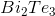?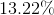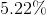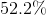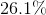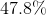Explanation:

The mass percentage of bismuth in the compound will be equal to the mass of bismuth in one mole of compound divided by the total molar mass of the compound.

Bismuth has a molar mass of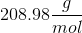. One mole of the compound would result in two moles of bismuth, a total of 417.96g.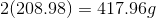Tellurium has a molar mass of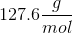. One mole of the compound would result in three moles of tellurium, a total of 382.8g.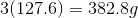Add the mass of bismuth and the mass of tellurium per mole to find the total molar mass.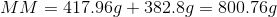Divide the mass of bismuth by the total molecular mass to find the percent by mass of bismuth in the compound.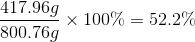### Example Question #2 : Mass

What is the percent by mass of carbon in acetic acid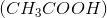?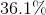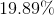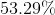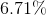Explanation:

The total molar mass of acetic acid is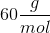.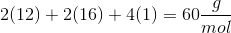Carbon contributes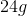total. Therefore, in a sample of 1 mole of acetic acid, there areof carbon. To find the percent by mass, we must divide the mass of carbon by the total molar mass of the compound.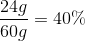### Example Question #3 : Mass

Calculate the percent by mass of each element in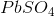.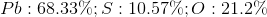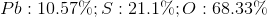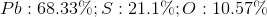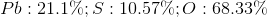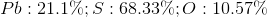Explanation:

The total molar mass of lead (II) sulfate is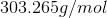. Lead contributes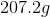, sulfur contributes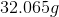, and oxygen contributes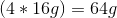.

The percent by mass of each element in the compound is found by dividing the mass contribution of that element by the total molar mass of the compound.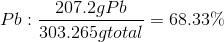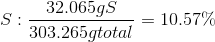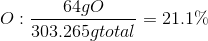### Example Question #4 : Mass

What percent by mass of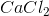is chlorine?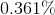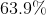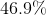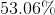Explanation:

The molar mass of calcium chloride is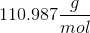. Chlorine has two moles per one mole of. Therefore, chloride contributes 70.9g to the total molecular mass.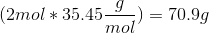To find the percent by mass of chlorine in calcium chloride, divide the contribution of chlorine by the total molar mass.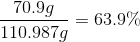### Example Question #5 : Mass

Calculate the percent by mass of each element in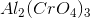.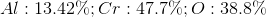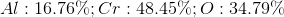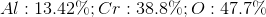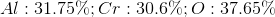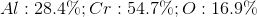Explanation:

The total mass of one mole of aluminum (II) chromate is calculated by: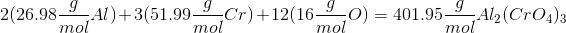Aluminum, chromate, and oxygen contribute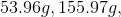and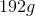respectively. Therefore, we can divide each contribution by the total molecular mass to determine percentages by mass.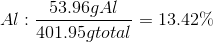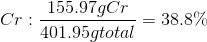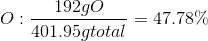### Example Question #6 : Mass

What is the percent by mass of hydrogen in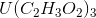?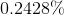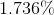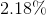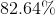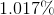Explanation:

The total mass ofis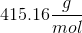and calculated by: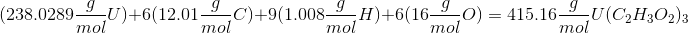You can then divide hydrogen's contribution by the total molecular mass in order to find the percent mass.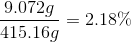### Example Question #7 : Mass

What percent of the overall mass is represented by the cation(s) in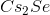?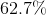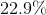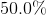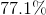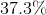Explanation:

The total molar mass ofis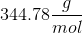. Cesium has a +1 charge when the compound is dissolved in solution and is, thus, the cation.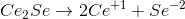Cesium contributes 265.8g to the total molecular mass.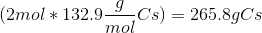We can find the percent by mass of cesium by dividing the mass of cesium by the total molecular mass.